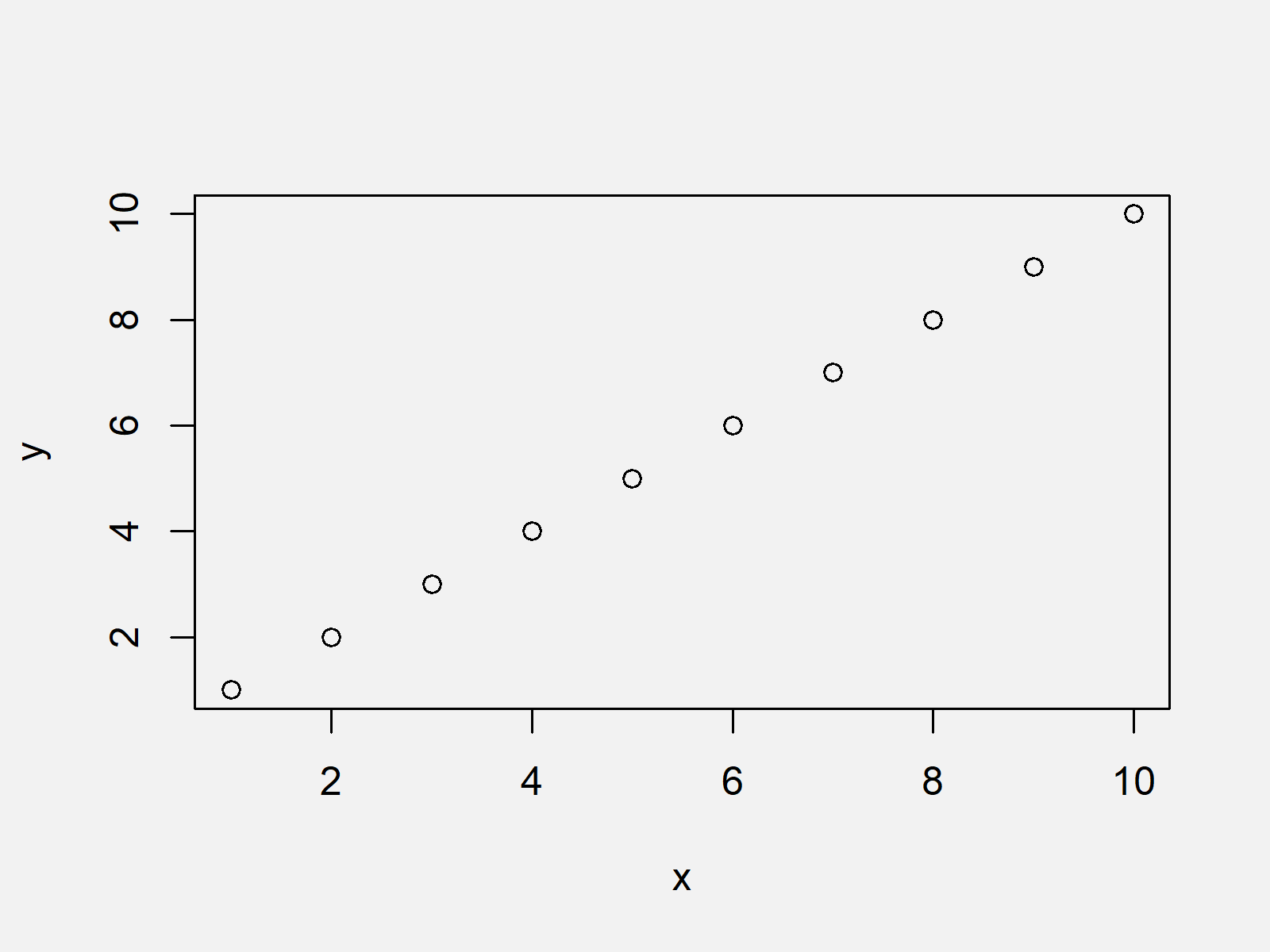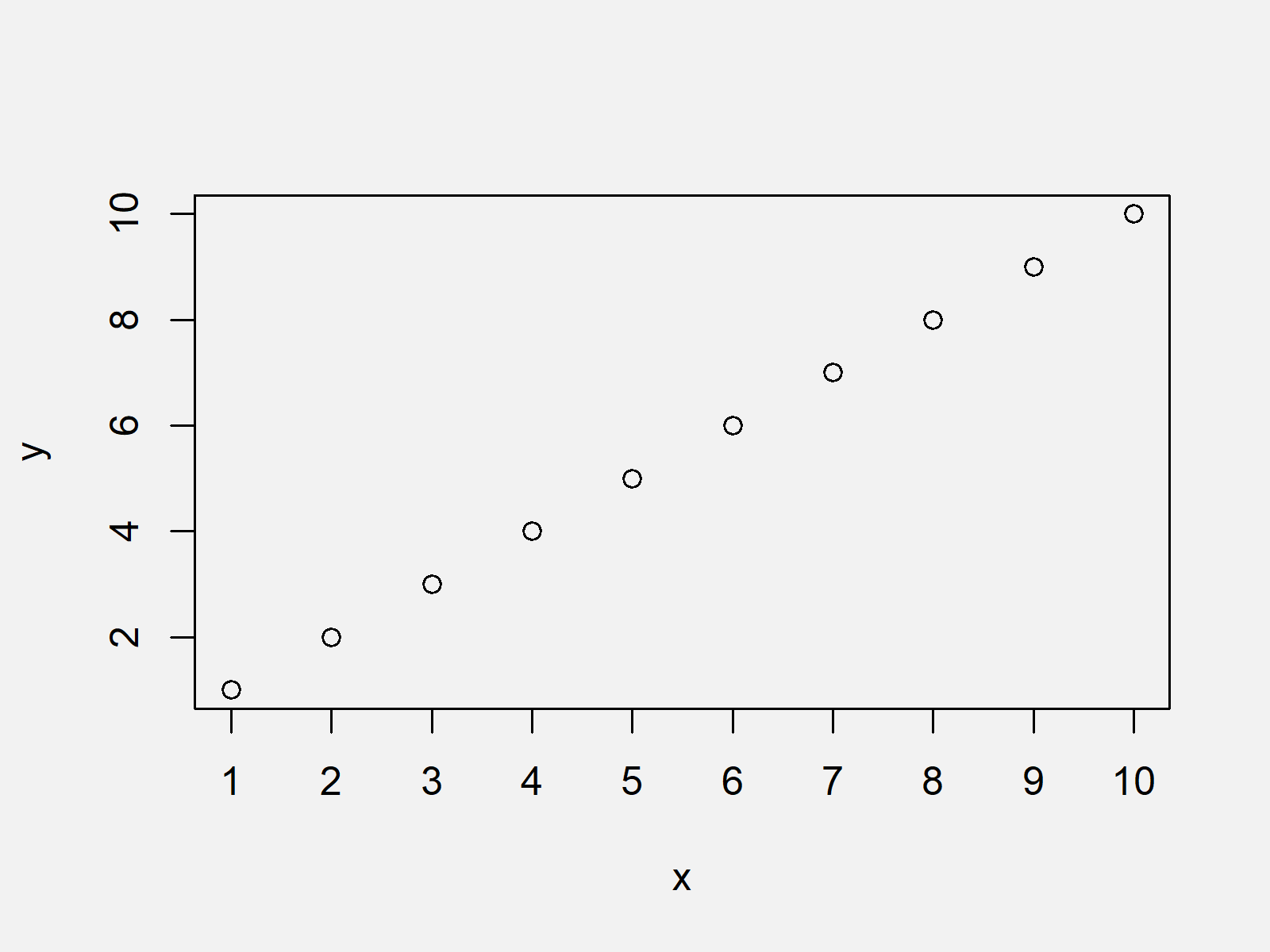# Draw Plot with Actual Values as Axis Ticks & Labels in R (2 Examples)

In this article, I’ll show how to use the real values of a plot as axis ticks in the R programming language.

It’s time to dive into the examples…

## Creating Example Data

We use the following data as basement for this R programming tutorial:

```x <- 1:10                          # Example data
y <- 1:10```

Our example data are two simple numeric vectors ranging from 1 to 10.

As next step, we can draw our data:

`plot(x, y)                         # Plot with default specifications`Figure 1 shows the output of the previous syntax: A scatterplot with default axes.

## Example 1: Drawing Plot with User-Defined Axis Using axis Function & at Argument

Example 1 illustrates how to modify axis values using the axis function in R.

For this, we first need to specify the xaxt argument of the plot function to be equal to “n”.

Then, we have to specify within the axis function at which position we want to draw our axis (1 stands for the x-axis) and at which values the axis ticks and labels should be shown (i.e. at = x).

```plot(x, y, xaxt = "n")             # Draw plot without axis
axis(1, at = x)                    # Applying axis function```The output of the previous R programming code is shown in Figure 2 – It shows a graph with manually specified x-axis ticks and labels that are equal to the actual numbers in our data.

## Example 2: Drawing Plot with User-Defined Axis Using axis Function & xaxp Argument

This Example shows an alternative to the R code of Example 1 that leads to the same output. In this example, we are using the xaxp argument of the axis function instead of the at argument:

```plot(x, y, xaxt = "n")             # Draw plot without axis
axis(1, xaxp = c(1, 10, 9))        # Applying axis function```Figure 3 shows the output of the previously shown R programming syntax – The same graphic as in Example 1.

## Video & Further Resources

Have a look at the following video which I have published on my YouTube channel. In the video, I show the topics of this article:

Please accept YouTube cookies to play this video. By accepting you will be accessing content from YouTube, a service provided by an external third party.If you accept this notice, your choice will be saved and the page will refresh.

In addition, you might want to read the other articles of this website. I have released numerous other articles on topics such as ggplot2, graphics in r, and labels.

Summary: On this page you learned how to specify axis ticks based on the actual data points shown in the plot in R. In case you have further questions, let me know in the comments section.

Subscribe to the Statistics Globe Newsletter